# stftmag2sig

Signal reconstruction from STFT magnitude

## Syntax

``x = stftmag2sig(s,nfft)``
``x = stftmag2sig(s,nfft,fs)``
``x = stftmag2sig(s,nfft,ts)``
``x = stftmag2sig(___,Name,Value)``
``[x,t,info] = stftmag2sig(___)``

## Description

example

````x = stftmag2sig(s,nfft)` returns a reconstructed time-domain real signal, `x`, estimated from the Short-Time Fourier Transform (STFT) magnitude, `s`, based on the Griffin-Lim algorithm. The function assumes `s` was computed using discrete Fourier transform (DFT) length `nfft`.```

example

````x = stftmag2sig(s,nfft,fs)` returns the reconstructed signal assuming that `s` was sampled at rate `fs`.```
````x = stftmag2sig(s,nfft,ts)` returns the reconstructed signal assuming that `s` was sampled with sample time `ts`.```
````x = stftmag2sig(___,Name,Value)` specifies additional options using name-value pair arguments. Options include, among others, the FFT window and the method to specify initial phases. These arguments can be added to any of the previous input syntaxes. For example, `'FrequencyRange','onesided','InitializePhaseMethod','random'` specifies that the signal is reconstructed from a one-sided STFT with random initial phases.```
````[x,t,info] = stftmag2sig(___)` also returns the time instants at which the signal is reconstructed and a structure that contains information about the reconstruction process.```

## Examples

collapse all

Consider 512 samples of a sinusoid with a normalized frequency of $\pi /60$ rad/sample and a DC value of 1. Compute the STFT of the signal.

```n = 512; x = cos(pi/60*(0:n-1)')+1; S = stft(x);```

Reconstruct the sinusoid from the magnitude of the STFT. Plot the original and reconstructed signals.

```xr = stftmag2sig(abs(S),size(S,1)); plot(x) hold on plot(xr,'--','LineWidth',2) hold off legend('Original','Reconstructed')```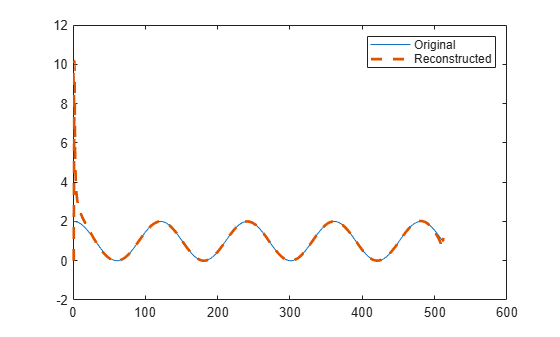Repeat the computation, but now pad the signal with zeros to decrease edge effects.

```xz = circshift([x; zeros(n,1)],n/2); Sz = stft(xz); xr = stftmag2sig(abs(Sz),size(Sz,1)); xz = xz(n/2+(1:n)); xr = xr(n/2+(1:n)); plot(xz) hold on plot(xr,'--','LineWidth',2) hold off legend('Original','Reconstructed')```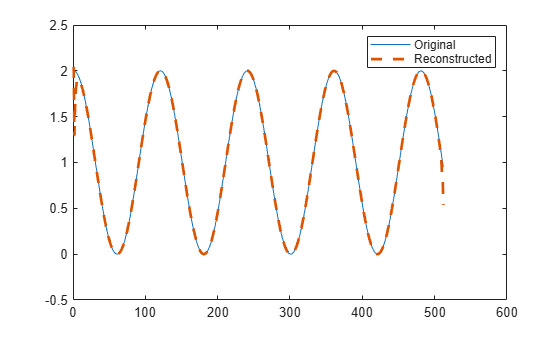Repeat the computation, but now decrease edge effects by assuming that `x` is a segment of a signal twice as long.

```xx = cos(pi/60*(-n/2:n/2+n-1)')+1; Sx = stft(xx); xr = stftmag2sig(abs(Sx),size(Sx,1)); xx = xx(n/2+(1:n)); xr = xr(n/2+(1:n)); plot(xx) hold on plot(xr,'--','LineWidth',2) hold off legend('Original','Reconstructed')```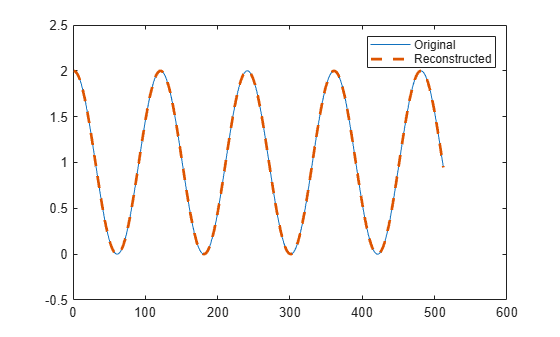Load an audio signal that contains two decreasing chirps and a wideband splatter sound. The signal is sampled at 8192 Hz. Plot the STFT of the signal. Divide the waveform into 128-sample segments and window the segments using a Hamming window. Specify 64 samples of overlap between adjoining segments and 1024 FFT points.

```load splat ty = (0:length(y)-1)/Fs; % To hear, type sound(y,Fs) wind = hamming(128); olen = 64; nfft = 1024; stft(y,Fs,'Window',wind,'OverlapLength',olen,'FFTLength',nfft)```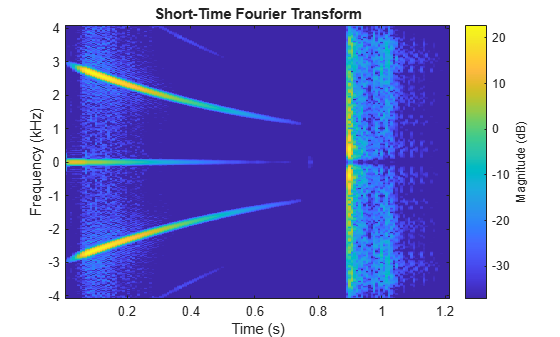Compute the magnitude and phase of the STFT.

```s = stft(y,Fs,'Window',wind,'OverlapLength',olen,'FFTLength',nfft); smag = abs(s); sphs = angle(s);```

Reconstruct the signal based on the magnitude of the STFT. Use the same parameters that you used to compute the STFT. By default, `stftmag2sig` initializes the phases to zero and uses 100 optimization iterations.

```[x,tx,info] = stftmag2sig(smag,nfft,Fs,'Window',wind,'OverlapLength',olen); % To hear, type sound(x,Fs)```

Plot the original and reconstructed signals. For better comparison, offset the reconstructed signal up and to the right.

```plot(ty,y,tx+500/Fs,x+1) legend('Original','Reconstructed','Location','best')```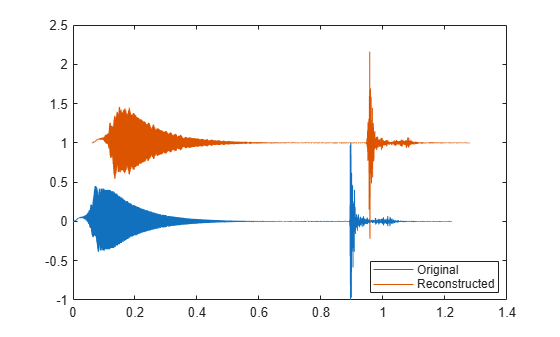Output the relative improvement toward convergence between the last two iterations.

`impr = info.Inconsistency`
```impr = 0.0424 ```

Improve the reconstruction by doubling the number of optimization iterations and setting the initial phases to the actual phases from the STFT. Plot the original and reconstructed signals. For better comparison, plot the negative of the reconstructed signal and offset it up and to the right.

```[x,tx,info] = stftmag2sig(smag,nfft,Fs,'Window',wind,'OverlapLength',olen, ... 'MaxIterations',200,'InitialPhase',sphs); % To hear, type sound(x,Fs) plot(ty,y,tx+500/Fs,-x+1) legend('Original','Reconstructed','Location','best')```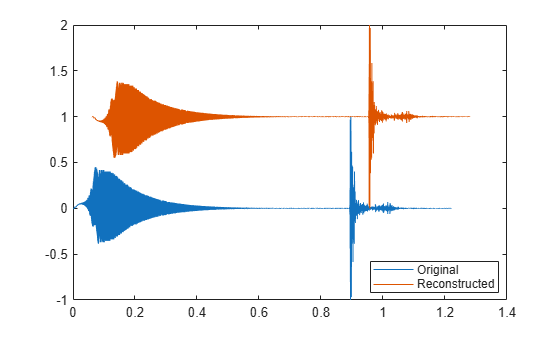Output the relative improvement toward convergence between the last two iterations.

`impr = info.Inconsistency`
```impr = 1.3874e-16 ```

## Input Arguments

collapse all

STFT magnitude, specified as a matrix. `s` must correspond to a single-channel, real-valued signal.

Example: `abs(stft(sin(pi/2*(0:255)),'FFTLength',128))` specifies the STFT magnitude of a sinusoid.

Example: `abs(stft(chirp(0:1/1e3:1,25,1,50)))` specifies the STFT magnitude of a chirp sampled at 1 kHz.

Data Types: `single` | `double`

Number of DFT points, specified as a positive integer scalar. This argument is always required.

Data Types: `single` | `double`

Sample rate, specified as a positive numeric scalar.

Sample time, specified as a `duration` scalar. Specifying `ts` is equivalent to setting a sample rate fs = 1/`ts`.

Example: `seconds(1)` is a `duration` scalar representing a 1-second time difference between consecutive signal samples.

Data Types: `duration`

### Name-Value Arguments

Specify optional comma-separated pairs of `Name,Value` arguments. `Name` is the argument name and `Value` is the corresponding value. `Name` must appear inside quotes. You can specify several name and value pair arguments in any order as `Name1,Value1,...,NameN,ValueN`.

Example: `'FrequencyRange','onesided','InitializePhaseMethod','random'` specifies that the signal is reconstructed from a one-sided STFT with random initial phases.

Inconsistency display option, specified as the comma-separated pair consisting of `'Display'` and a logical value. If this option is set to `true`, then `stftmag2sig` displays the normalized inconsistency after every 20 optimization iterations, and it also displays stopping information at the end of the run.

Data Types: `logical`

Frequency range of STFT magnitude, specified as the comma-separated pair consisting of `'FrequencyRange'` and `'centered'`, `'twosided'`, or `'onesided'`.

• `'centered'` — Treat `s` as the magnitude of a two-sided, centered STFT. If `nfft` is even, then `s` is taken to have been computed over the interval (–π, π] rad/sample. If `nfft` is odd, then `s` is taken to have been computed over the interval (–π, π) rad/sample. If you specify time information, then the intervals are (–fs, fs/2] cycles/unit time and (–fs, fs/2) cycles/unit time, respectively, where fs is the sample rate.

• `'twosided'` — Treat `s` as the magnitude of a two-sided STFT computed over the interval [0, 2π) rad/sample. If you specify time information, then the interval is [0, fs) cycles/unit time.

• `'onesided'` — Treat `s` as the magnitude of a one-sided STFT. If `nfft` is even, then `s` is taken to have been computed over the interval [0, π] rad/sample. If `nfft` is odd, then `s` is taken to have been computed over the interval [0, π) rad/sample. If you specify time information, then the intervals are [0, fs/2] cycles/unit time and [0, fs/2) cycles/unit time, respectively, where fs is the sample rate.

Data Types: `char` | `string`

Inconsistency tolerance of reconstruction process, specified as the comma-separated pair consisting of `'InconsistencyTolerance'` and a positive scalar. The reconstruction process stops when the Normalized Inconsistency is lower than the tolerance.

Data Types: `single` | `double`

Phase initialization, specified as the comma-separated pair consisting of `'InitializePhaseMethod'` and `'zeros'` or `'random'`. Specify only one of `'InitializePhaseMethod'` or `'InitialPhase'`.

• `'zeros'` — The function initializes the phases as zeros.

• `'random'` — The function initializes the phases as random numbers distributed uniformly in the interval [–π, π].

Data Types: `char` | `string`

Initial phases, specified as the comma-separated pair consisting of `'InitialPhase'` and a real numeric matrix in the range [–π, π]. The matrix must have the same size as `s`. Specify only one of `'InitializePhaseMethod'` or `'InitialPhase'`.

Example: `angle(stft(randn(1000,1)))` specifies the phases of the short-time Fourier transform of a random signal.

Example: `2*pi*(rand(size(stft(randn(1000,1))))-1/2)` specifies a matrix of random phases distributed uniformly in the interval [–π, π]. The matrix has the same size as the short-time Fourier transform of a random signal.

.

Data Types: `single` | `double`

Input time dimension, specified as the comma-separated pair consisting of `'InputTimeDimension'` and `'acrosscolumns'` or `'downrows'`.

• `'acrosscolumns'` — The function assumes that the time dimension of `s` is across the columns and the frequency dimension is down the rows.

• `'downrows'` — The function assumes that the time dimension of `s` is down the rows and the frequency dimension is across the columns.

Data Types: `char` | `string`

Maximum number of optimization iterations, specified as the comma-separated pair consisting of `'MaxIterations'` and a positive integer scalar. The reconstruction process stops when the number of iterations is greater than `'MaxIterations'`.

Data Types: `single` | `double`

Signal reconstruction algorithm, specified as the comma-separated pair consisting of `'Method'` and one of these:

• `'gla'` — The original reconstruction algorithm, proposed by Griffin and Lim and described in .

• `'fgla'` — A fast Griffin-Lim algorithm proposed by Perraudin, Balazs, and Søndergaard and described in .

• `'legla'` — A fast algorithm proposed by Le Roux, Kameoka, Ono, and Sagayama and described in .

Data Types: `char` | `string`

Number of overlapped samples between adjoining segments, specified as the comma-separated pair consisting of `'OverlapLength'` and a positive integer smaller than the length of `'Window'`. Successful signal reconstruction requires `'OverlapLength'` to match the number of overlapped segments used to generate the STFT magnitude. If you omit `'OverlapLength'` or specify it as empty, it is set to the largest integer less than or equal to 75% of the window length, which is 96 samples for the default Hann window.

Data Types: `double` | `single`

Truncation order for `'legla'` update rule, specified as the comma-separated pair consisting of `'TruncationOrder'` and a positive integer. This argument applies only when `'Method'` is set to `'legla'` and controls the number of phase values updated in each iteration of that method. If not specified, `'TruncationOrder'` is determined using an adaptive algorithm.

Data Types: `single` | `double`

Update parameter for the fast Griffin-Lim algorithm, specified as the comma-separated pair consisting of `'UpdateParameter'` and a positive scalar. This argument applies only when `'Method'` is set to `'fgla'` and specifies the parameter for that method's update rule.

Data Types: `single` | `double`
Complex Number Support: Yes

Spectral window, specified as the comma-separated pair consisting of `'Window'` and a vector. Successful signal reconstruction requires `'Window'` to match the window used to generate the STFT magnitude. If you do not specify the window or specify it as empty, the function uses a periodic Hann window of length 128. The length of `'Window'` must be greater than or equal to 2.

For a list of available windows, see Windows.

Example: `hann(128,'periodic')` and `(1-cos(2*pi*(128:-1:1)'/128))/2` both specify the default window used by `stftmag2sig`.

Data Types: `double` | `single`

## Output Arguments

collapse all

Reconstructed time-domain signal, returned as a vector.

Time instants at which the signal is reconstructed, returned as a vector.

Reconstruction process information, returned as a structure containing these fields:

• `ExitFlag` — Termination flag.

• A value of `0` indicates the algorithm stopped when it reached the maximum number of iterations.

• A value of `1` indicates the algorithm stopped when it met the relative tolerance.

• `NumIterations` — Total number of iterations.

• `Inconsistency` — Average relative improvement toward convergence between the last two iterations.

• `ReconstructedPhase` — Reconstructed phase at the last iteration.

• `ReconstructedSTFT` — Reconstructed short-time Fourier transform at the last iteration.

collapse all

### Short-Time Fourier Transform

The short-time Fourier transform (STFT) is used to analyze how the frequency content of a nonstationary signal changes over time.

The STFT of a signal is calculated by sliding an analysis window of length $M$ over the signal and calculating the discrete Fourier transform of the windowed data. The window hops over the original signal at intervals of $R$ samples. Most window functions taper off at the edges to avoid spectral ringing. If a nonzero overlap length $L$ is specified, overlap-adding the windowed segments compensates for the signal attenuation at the window edges. The DFT of each windowed segment is added to a matrix that contains the magnitude and phase for each point in time and frequency. The number of columns in the STFT matrix is given by

`$k=⌊\frac{{N}_{x}-L}{M-L}⌋,$`

where ${N}_{x}$ is the length of the original signal $x\left(n\right)$ and the ⌊⌋ symbols denote the floor function. The number of rows in the matrix equals NDFT, the number of DFT points, for centered and two-sided transforms and NDFT/2⌋ + 1 for one-sided transforms.

The STFT matrix is given by $X\left(f\right)=\left[\begin{array}{ccccc}{X}_{1}\left(f\right)& {X}_{2}\left(f\right)& {X}_{3}\left(f\right)& \cdots & {X}_{k}\left(f\right)\end{array}\right]$ such that the $m$th element of this matrix is

`${X}_{m}\left(f\right)=\sum _{n=-\infty }^{\infty }x\left(n\right)g\left(n-mR\right){e}^{-j2\pi fn},$`

where

• $g\left(n\right)$ — Window function of length $M$.

• ${X}_{m}\left(f\right)$ — DFT of windowed data centered about time $mR$.

• $R$ — Hop size between successive DFTs. The hop size is the difference between the window length $M$and the overlap length $L$.

The magnitude squared of the STFT yields the `spectrogram` representation of the power spectral density of the function.### Normalized Inconsistency

The normalized inconsistency measures the improvement toward convergence of the reconstruction process in successive optimization iterations.

The normalized inconsistency is defined as

`$\text{Inconsistency}=\frac{‖\mathrm{STFT}\left(\mathrm{ISTFT}\left({s}_{\text{est}}\right)\right)-{s}_{\text{est}}‖}{‖{s}_{\text{est}}‖},$`

where sest is the complex short-time Fourier transform estimated at each iteration, the brackets denote the matrix norm, STFT denotes the short-time Fourier transform, and ISTFT denotes its inverse. `stftmag2sig` uses the MATLAB® function `norm` to compute matrix norms. For more information about the STFT and its inverse, see Short-Time Fourier Transform and Inverse Short-Time Fourier Transform.

 Griffin, Daniel W., and Jae S. Lim. "Signal Estimation from Modified Short-Time Fourier Transform." IEEE Transactions on Acoustics, Speech, and Signal Processing. Vol. 32, Number 2, April 1984, pp. 236–243. https://doi.org/10.1109/TASSP.1984.1164317.

 Perraudin, Nathanaël, Peter Balazs, and Peter L. Søndergaard. "A Fast Griffin-Lim Algorithm." In 2013 IEEE Workshop on Applications of Signal Processing to Audio and Acoustics, New Paltz, NY, October 20–23, 2013. https://doi.org/10.1109/WASPAA.2013.6701851.

 Le Roux, Jonathan, Hirokazu Kameoka, Nobutaka Ono, and Shigeki Sagayama. "Fast Signal Reconstruction from Magnitude STFT Spectrogram Based on Spectrogram Consistency." In Proceedings of the 13th International Conference on Digital Audio Effects (DAFx-10), Graz, Austria, September 6–10, 2010.